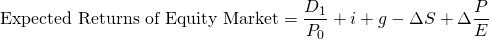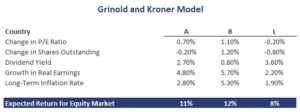# Grindold and Kroner model

The Grinold and Kroner model (GK model) is an equity market performance forecasting model. Thus, the model can be used to forecast the equity risk premium. The Grinold and Kroner equity risk premium model was proposed by Richard Grinold and Kenneth Kroner in 2002 in a paper called “The Equity Risk Premium”.

We discuss the Grinold and Kroner model formula and discuss a numerical example that can easily be implemented using an Excel spreadsheet. The spreadsheet is available at the bottom of the page.

## Grinold and Kroner model components

The best was to discuss the Grinold and Kroner model is by looking at the components. Let’s have a look at the Grinold and Kroner formulawhere D1 is the dividend in next period, P0 is the current price, i is the expected rate of inflation, g is the real growth rate in earnings, ΔS is the change in the number of shares outstanding, and Δ(P/E) is the change in the P/E ratio.

Now, let’s discuss the components in more detail. Expected inflation plus the real growth rate together makes up the nominal growth rate. Thus, the nominal growth rate is the first element we need. Next, the change in shares outstanding is subtracted. That is because an increase in the number of shares outstanding means that that dividends have to be distributed over more shares. This decreases the expected return and this variable is sometimes also referred to as the repurchase yield. Finally, the change in the P/E ratio positively influences the expected return.

## Grinold Kroner model example

Next, let’s turn to a simple numerical example to illustrate how to calculate the expected equity risk premium using the GK model. In particular, let’s calculate the market risk premium for three different countries A, B, and C. The results and the calculation are reported belowThe Excel spreadsheet we used to calculate the above results is available at the bottom of the page.

## Summary

We discussed the Grinold and Kroner equity risk premium model and its components. The model is commonly used by analysts to forecast the risk premium.

### Grinold and Kroner model calculator

Want to value equities using the GK model in Excel? Download the Excel file: Grinold and Kroner Model template